Algebra facts for kids

Kids Encyclopedia Facts - Homework Help

Algebra is a part of mathematics (often called math in the United States and maths in the United Kingdom ). It uses variables to represent a value that is not yet known. When an equals sign (=) is used, this is called an equation. A very simple equation using a variable is: 2 + 3 = x In this example, x = 5, or it could also be said, "x is five". This is called solving for x.

Besides equations, there are inequalities (less than and greater than). A special type of equation is called the function. This is often used in making graphs.

Algebra can be used to solve real problems because the rules of algebra work in real life and numbers can be used to represent the values of real things. Physics, engineering and computer programming are areas that use algebra all the time. It is also useful to know in surveying, construction and business, especially accounting.

People who do algebra need to know the rules of numbers and mathematic operations used on numbers, starting with adding, subtracting, multiplying, and dividing. More advanced operations involve exponents, starting with squares and square roots. Many of these rules can also be used on the variables, and this is where it starts to get interesting.

Algebra was first used to solve equations and inequalities. Two examples are linear equations (the equation of a line, y=mx+b) and quadratic equations, which has variables that are squared (power of two, a number that is multiplied by itself, for example: 2*2, 3*3, x*x). How to factor polynomials is needed for quadratic equations.

History

Early forms of algebra were developed by the Babylonians and the Greeks. However the word "algebra" is a Latin form of the Arabic word Al-Jabr ("casting") and comes from a mathematics book Al-Maqala fi Hisab-al Jabr wa-al-Muqabilah, ("Essay on the Computation of Casting and Equation") written in the 9th century by a Persian mathematician, Muhammad ibn Mūsā al-Khwārizmī, who was a Muslim born in Khwarizm in Uzbekistan. He flourished under Al-Ma'moun in Baghdad, Iraq through 813-833 AD, and died around 840 AD. The book was brought into Europe and translated into Latin in the 12th century. The book was then given the name 'Algebra'. (The ending of the mathematician's name, al-Khwarizmi, was changed into a word easier to say in Latin, and became the English word algorithm).

Examples

Here is a simple example of an algebra problem:

Sue has 12 candies, Ann has 24 candies. They decide to share so that they have the same number of candies.

These are the steps you can use to solve the problem:

1. To have the same number of candies, Ann has to give some to Sue. Let x represent the number of candies Ann gives to Sue.
2. Sue's candies, plus x, must be the same as Ann's candies minus x. This is written as: 12 + x = 24 - x
3. Subtract 12 from both sides of the equation. This gives: x = 12 - x. (What happens on one side of the equals sign must happen on the other side too, for the equation to still be true. So in this case when 12 was subtracted from both sides, there was a middle step of 12 + x - 12 = 24 - x - 12. After a person is comfortable with this, the middle step is not written down.)
4. Add x to both sides of the equation. This gives: 2x = 12
5. Divide both sides of the equation by 2. This gives x = 6. The answer is six. If Ann gives Sue 6 candies, they will have the same number of candies.
6. To check this, put 6 back into the original equation wherever x was: 12 + 6 = 24 - 6
7. This gives 18=18, which is true. They both now have 18 candies.

With practice, algebra can be used when faced with a problem that is too hard to solve any other way. Problems such as building a freeway, designing a cell phone, or finding the cure for a disease all require algebra.

Writing algebra

As in most parts of mathematics, adding z to y (or y plus z) is written as y + z. Subtracting z from y (or y minus z) is written as y − z. Dividing y by z (or y over z: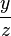$y \over z$) is written as y ÷ z or y/z. y/z is more commonly used.

In algebra, multiplying y by z (or y times z) can be written in 4 ways: y × z, y * z, y·z, or just yz. The multiplication symbol "×" is usually not used, because it looks too much like the letter x, which is often used as a variable. Also, when multiplying a larger expression, parentheses can be used: y (z+1).

When we multiply a number and a letter in algebra, we write the number in front of the letter: 5 × y = 5y. When the number is 1, then the 1 is not written because 1 times any number is that number (1 × y = y) and so it is not needed.

Functions and Graphs

An important part of algebra is the study of functions, since functions often appear in equations that we are trying to solve. A function is like a box you can put a number or numbers into and get a certain number out. When using functions, graphs can be powerful tools in helping us to study the solutions to equations.

A graph is a picture that shows all the values of the variables that make the equation or inequality true. Usually this is easy to make when there are only one or two variables. The graph is often a line, and if the line does not bend or go straight up-and-down it can be described by the basic formula y = mx + b. The variable b is the y-intercept of the graph (where the line crosses the vertical axis) and m is the slope or steepness of the line. This formula applies to the coordinates of a graph, where each point on the line is written (x, y).

In some math problems like the equation for a line, there can be more than one variable (x and y in this case). To find points on the line, one variable is changed. The variable that is changed is called the "independent" variable. Then the math is done to make a number. The number that is made is called the "dependent" variable. Most of the time the independent variable is written as x and the dependent variable is written as y, for example, in y = 3x + 1. This is often put on a graph, using an x axis (going left and right) and a y axis (going up and down). It can also be written in function form: f(x) = 3x + 1. So in this example, we could put in 5 for x and get y = 16. Put in 2 for x would get y=7. And 0 for x would get y=1. So there would be a line going thru the points (5,16), (2,7), and (0,1) as seen in the graph to the right.

If x has a power of 1, it is a straight line. If it is squared or some other power, it will be curved. If it uses an inequality (< or >), then usually part of the graph is shaded, either above or below the line.

Rules of algebra

In algebra, there are a few rules that can be used for further understanding of equations. These are called the rules of algebra. While these rules may seem senseless or obvious, it is wise to understand that these properties do not hold throughout all branches of mathematics. Therefore, it will be useful to know how these axiomatic rules are declared, before taking them for granted. Before going on to the rules, reflect on two definitions that will be given.

1. Opposite - the opposite of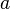$a$ is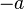$-a$.
2. Reciprocal - the reciprocal of$a$ is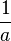$\frac{1}{a}$.

Rules

'Commutative' means that a function has the same result if the numbers are swapped around. In other words, the order of the terms in an equation do not matter. When the operator of two terms is an addition, the 'commutative property of addition' is applicable. In algebraic terms, this gives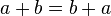$a + b = b + a$.

Note that this does not apply for subtraction! (i.e.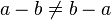$a - b \ne b - a$)

Commutative property of multiplication

When the operator of two terms is an multiplication, the 'commutative property of multiplication' is applicable. In algebraic terms, this gives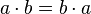$a \cdot b = b \cdot a$.

Note that this does not apply for division! (i.e.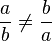$\frac{a}{b} \ne \frac{b}{a}$, when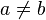$a \neq b$)

'Associative' refers to the grouping of numbers. The associative property of addition implies that, when adding three or more terms, it doesn't matter how these terms are grouped. Algebraically, this gives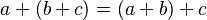$a + (b + c) = (a + b) + c$. Note that this does not hold for subtraction, e.g.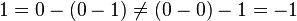$1 = 0 - (0 - 1) \neq (0 - 0) - 1 = -1$ (see the distributive property).

Associative property of multiplication

The associative property of multiplication implies that, when multiplying three or more terms, it doesn't matter how these terms are grouped. Algebraically, this gives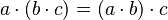$a \cdot (b \cdot c) = (a \cdot b) \cdot c$. Note that this does not hold for division, e.g.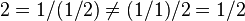$2 = 1/(1/2) \neq (1/1)/2 = 1/2$.

Distributive property

The distributive property states that the multiplication of a number by another term can be distributed. For instance: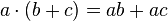$a \cdot (b + c) = ab + ac$. (Do not confuse this with the associative properties! For instance,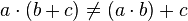$a \cdot (b + c) \ne (a \cdot b) + c$.)

'Identity' refers to the property of a number that it is equal to itself. In other words, there exists an operation of two numbers so that it equals the variable of the sum. The additive identity property states that the sum of any number and 0 is that number: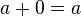$a + 0 = a$. This also holds for subtraction: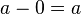$a - 0 = a$.

Multiplicative identity property

The multiplicative identity property states that the product of any number and 1 is that number: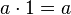$a \cdot 1 = a$. This also holds for division: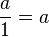$\frac{a}{1} = a$.

The additive inverse property is somewhat like the opposite of the additive identity property. When an operation is the sum of a number and its opposite, and it equals 0, that operation is a valid algebraic operation. Algebraically, it states the following: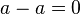$a - a = 0$. Additive inverse of 1 is (-1).

Multiplicative inverse property

The multiplicative inverse property entails that when an operation is the product of a number and its reciprocal, and it equals 1, that operation is a valid algebraic operation. Algebraically, it states the following: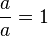$\frac{a}{a} = 1$. Multiplicative inverse of 2 is 1/2.